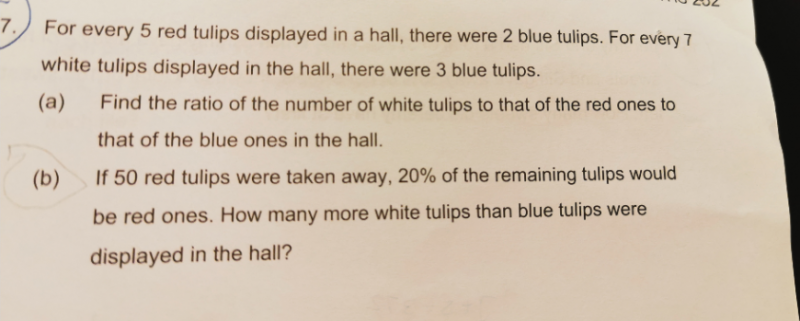# QuestionHi,

Need help on this Qns.

Thanks 😊

a)

 White Red Blue 5 units 2 units x 3 7 units 3 units x 2 14 units 15 units 6 units

14 : 15 : 6

b)

 White Red Blue Total Before 14 units 15 units 6 units 35 units After 14 units 15 units – 50 6 units 35 units – 50

We know that after 5o red tulips were taken away, the red tulips made up 20% (1/5) of the total number of tulips. This means that if we multiply the quantity of red tulips by 5, it should equal the total number of tulips.

(15 units – 50) x 5 = 35 units – 50
75 units – 250 = 35 units – 50
75 units – 35 units = 250 – 50
40 units = 200
1 unit = 5

White tulips → 14 x 5
= 70

Blue tulips → 6 x 5
= 30

70 – 30 = 40 more white tulips than blue tulips

0 Replies 0 Likes